Reach Us+44-1522-440391
Exact Tests for the Weak Causal Null Hypothesis on a Binary Out come in Randomized Trials | OMICS International
Journal of Biometrics & Biostatistics

Like us on:

All submissions of the EM system will be redirected to Online Manuscript Submission System. Authors are requested to submit articles directly to Online Manuscript Submission System of respective journal.

# Exact Tests for the Weak Causal Null Hypothesis on a Binary Out come in Randomized Trials

Yasutaka Chiba*

Clinical Research Center, Kinki University Hospital, Japan

*Corresponding Author:
Yasutaka Chiba
Clinical Research Center
Kinki University Hospital, Japan
Tel: +81-72-366-0221
Fax: +81-72-368-1193
E-mail: [email protected]

Received Date: July 27, 2015; Accepted Date: August 18, 2015; Published Date: August 25, 2015

Citation: Chiba Y (2015) Exact Tests for the Weak Causal Null Hypothesis on a Binary Outcome in Randomized Trials. J Biom Biostat 6: 244. doi:10.4172/2155-6180.1000244

Copyright: © 2015 Chiba Y. This is an open-access article distributed under the terms of the Creative Commons Attribution License, which permits unrestricted use, distribution, and reproduction in any medium, provided the original author and source are credited.

Visit for more related articles at Journal of Biometrics & Biostatistics

#### Abstract

There are two principal exact tests for evaluation of data in two-by-two contingency tables: the tests of Fisher and Barnard. The latter cannot be a hypothesis test for the causal null hypothesis unless exchangeability can be assumed. Fisher’s exact test is a hypothesis test for the sharp causal null hypothesis (i.e., that there is no effect for all individuals), but not for the weak causal null hypothesis (i.e., that the true risk difference is zero). Rejection of the sharp causal null hypothesis does not mean that the weak causal null hypothesis is rejected (i.e., that the true risk difference is not zero). In this article, we provide exact tests for the weak causal null hypothesis, in the absence of any assumption, in the context of randomized trials. Using the concept of principal stratification, which considers four types of subjects to define four principal strata, we derive an unconditional exact test, for which neither marginal total is fixed, and a conditional exact test, for which one marginal total is fixed. In addition, we show that Fisher’s exact test can be a hypothesis test for the weak causal null hypothesis when monotonicity can be assumed. The derived exact tests are extended to hypothesis testing for non-inferiority trials and to construct confidence intervals linking to the exact tests. The derived exact tests and confidence intervals are illustrated using data from two clinical trials.

#### Keywords

Complete (simple) randomization; Conditional and unconditional exact tests; Exchangeability; Monotonicity; Potential outcome; Principal stratification

#### Introduction

We consider a randomized trial, in which subjects are assigned randomly to receive one of two experimental treatments, and each subject is classified as either a responder or a non-responder. In such a setting, data are summarized in a two-by-two contingency table, and hypothesis testing is performed to test the equality of the response proportions of the two groups.

Ninety years ago, Fisher [1,2] developed an exact test, which is often referred to as Fisher’s exact test. This test is a hypothesis test for the sharp, but not the weak, causal null hypothesis. Because rejection of the sharp causal null hypothesis does not imply that the weak causal null hypothesis is rejected, the true risk difference may still be zero even when the sharp causal null hypothesis is rejected by Fisher’s exact test.

Twenty years later, Barnard [3-5] developed another exact test, which is sometimes referred to as Barnard’s exact test. This test utilizes the product of two binomial probabilities, and it has advantages over Fisher’s exact test in that it can be more powerful for moderate to small samples [6,7]. Nevertheless, Barnard’s exact test cannot be a hypothesis test for the causal null hypothesis unless exchangeability can be assumed.

In this article, we provide exact tests for the weak causal null hypothesis without requiring any assumption (such as exchangeability) in the context of randomized trials, using the concept of principal stratification. To our knowledge, such an exact test has not been developed. First, an unconditional exact test for which neither marginal total is fixed is derived; then, a conditional exact test for which one marginal total is fixed is derived. In addition, we show that Fisher’s exact test can be a hypothesis test for the weak causal null hypothesis when monotonicity can be assumed. The derived exact tests are extended to hypothesis testing for non-inferiority trials and to construct confidence intervals linking to the exact tests. The derived exact tests and confidence intervals are illustrated using data from two clinical trials.

#### Notation and Principal Stratification Approach

Throughout this article, we denote X as the assigned treatment; X=1 if a subject was assigned to the treatment group, and X=0 if assigned to the control group. Y denotes the binary outcome; Y=1 if the event occurred, and Y=0 if it did not. The results from a randomized trial are summarized in a two-by-two contingency table as shown in Table 1, where a, b, c, d, and n are the numbers of subjects.

Event
Group Occurred (Y=1) Not occurred (Y=0) Total
Treatment (X=1) a b a+b
Control (X=0) c d c+d
Total a+c b+d n

Table 1: Two-by-two contingency table obtained from a randomized trial, where a, b, c, d, and n indicate the numbers of subjects

For each subject, it is also possible to consider the potential outcomes [8-10], which correspond to the outcomes of the subject had he/she been in the other group of the trial. Y(x) denotes the potential outcome for each subject under X=x. Then, Pr(Y(1)=1) represents a potential response proportion if all subjects are assigned to the treatment group, and Pr(Y(0)=1) represents a potential response proportion if all subjects are assigned to the control group. Using the potential outcome, the null hypothesis that the potential response proportions of each group are equal can be defined as

H0: Pr(Y(1)=1)=Pr(Y(0)=1).

This null hypothesis is referred to as the weak causal null hypothesis , and implies that two different treatment statuses from one population are compared.

Here, we apply the principal stratification approach . This approach considers the following four types of subjects to define the four principal strata:

(i) Individuals who would suffer the event regardless of the assigned treatment group; i.e., (Y(1), Y(0))=(1, 1).

(ii) Individuals who would suffer the event if assigned to the treatment group, but would not suffer the event if assigned to the control group; i.e., (Y(1), Y(0))=(1, 0).

(iii) Individuals who would not suffer the event if assigned to the treatment group, but would suffer the event if assigned to the control group; i.e., (Y(1), Y(0))=(0, 1).

(iv) Individuals who would not suffer the event regardless of the assigned treatment group; i.e., (Y(1), Y(0))=(0, 0).

These four types are summarized in Table 2, and all subjects belong to one of these four types.

Type Principal stratum Event under
the treatment group
Event under
the control group
(i) (Y(1), Y(0))=(1, 1) Yes Yes
(ii) (Y(1), Y(0))=(1, 0) Yes No
(iii) (Y(1), Y(0))=(0, 1) No Yes
(iv) (Y(1), Y(0))=(0, 0) No No

Table 2: Principal strata: “Yes” denotes that a subject would suffer the event, and “No” denotes that a subject would not suffer the event.

Let nst denote the number of subjects with (Y(1), Y(0))=(s, t), where s, t=0, 1. Although the value of nst cannot be determined from the observed data, we can nevertheless express the weak causal null hypothesis by using nst. If all subjects are assigned to the treatment group (X=1), Pr(Y(1)=1)=(n11+n10)/n because only subjects with type (i) or (ii) would suffer the event (Table 2). Likewise, if all subjects are assigned to the control group (X=0), Pr(Y(0)=1)=(n11+n01)/n because only subjects with type (i) or (iii) would suffer the event. Thus, using the concept of the principal stratification, the weak causal null hypothesis can be expressed as n10=n01 from Pr(Y(1)=1)=Pr(Y(0)=1).

#### Proposed Exact Tests

Using the above notation, we derive an unconditional exact test for the weak causal null hypothesis n10=n01 in the context of randomized trials with complete (or equally simple) randomization, and apply it to derive a conditional exact test.

Unconditional exact test

When the random assignment is conducted by the ratio of 1:r, we assume that subjects are assigned as in Table 3 under the null hypothesis; i.e., of the nstsubjects, nst,1 subjects are assigned to the treatment group (X=1) with the probability of 1/(1+r) and nst,0 subjects are assigned to the control group (X=0) with the probability of r/(1+r). As each subject is independently assigned, we form the following probability:

Event
Group Occurred (Y=1) Not occurred (Y=0) Total
Treatment (X=1) n11,1+n10,1 n00,1+n01,1 n11,1+n10,1+n01,1+n00,1
Control (X=0) n11,0+n01,0 n00,0+n10,0 n11,0+n10,0+n01,0+n00,0
Total n11+n10,1+n01,0 n00+n01,1+n10,0 n

Table 3: Two-by-two contingency table with the numbers for the four types of subjects defining the four principal strata.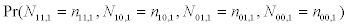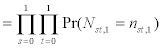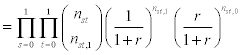(1)

where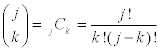, and the following set of conditions is required:

Set of conditions 1: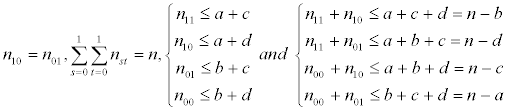The first condition is the null hypothesis and the second is the total number of subjects. The last two conditions are needed such that the numbers of subjects in principal strata under the null hypothesis, (n11, n10, n01, n00), do not contradict the observed data in Table 1; i.e., Table 3 is equal to Table 1 under at least one combination of nst,1 and nst,0. If (n11, n10, n01, n00) does contradict the observed data, subjects in the principal strata can no longer be the same sample as the subjects in the observed data. These conditions are derived from Tables 1 and 3 as follows; e.g., n11 ≤ a+c is derived from

a+c

=(n11,1+n10,1)+(n11,0+n01,0)

=n11+n10,1+n01,0,

and n11+n10 ≤ a+c+d is derived from

a+c+d

=(n11,1+n10,1)+(n11,0+n01,0)+(n00,0+n10,0)

=n11+n10+n01,0+n00,1.

The other inequalities are derived in a similar manner.

Here, we focus on the risk difference as the effect measure. The risk difference estimated from the observed data is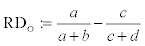from Table 1, and the risk difference under the null hypothesis is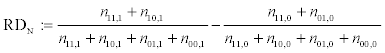from Table 3. We consider only the case of RDO ≤ 0 in this article, but the following methods can easily be applied to the case of RDO ≥ 0. For RDO ≤ 0, the one-sided p-value is defined as the probability that RDN is equal to or smaller than RDO if the same trial is conducted repeatedly under the null hypothesis. Therefore, Equation (1) yields the following one-sided p-value under a combination of (n11, n10, n01, n00) satisfying set of conditions 1: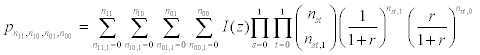where I(z)=1 if z ≤ 0 and I(z)=0 if z>0 with z := RDN-RDO. Note that for cases in which one denominator in RDN is 0, we set the indicator to I(z)=1. Although this setting of the indicator yields larger p-values than setting to I(z)=0, the substantial effect will be trivial because the probability that either nst,1 or nst,0 is 0 for all s and t is very small.

Unfortunately, this calculation of the p-value yields plural p-values corresponding to the number of combinations of (n11, n10, n01, n00), and then we cannot yield a p-value immediately. A method to deal with such a problem is to calculate the p-values for all possible combinations of (n11, n10, n01, n00) and choose the maximum value . Such a method may make the result of the hypothesis testing conservative. Using this method, we define the unconditional exact p-value based on the principal stratification as follows:

p sup={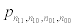:n11, n10, n01, n00 satisfying set of conditions 1}.

We note that neither marginal total is fixed for the unconditional exact test.

Conditional exact test

While the unconditional exact test does not fix the numbers of subjects assigned to the two groups, the conditional exact test does. Therefore, we consider a conditional probability on ΣsΣtNst,1ΣsΣtnst,1(=a+b) instead of Equation (1). This conditional probability can be expressed as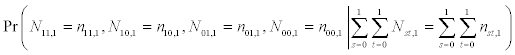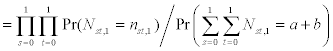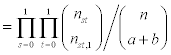where the following conditions are required:

Set of conditions 2:

Set of conditions 1 plus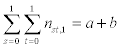Consequently, we can define the conditional exact p-value based on the principal stratification as follows: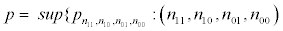satisfying set of conditions 2}

with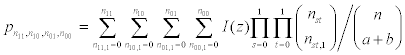(3)

#### Other Exact Tests

Here, we discuss assumptions for Fisher’s and Barnard’s exact tests being hypothesis tests for the weak causal null hypothesis.

Fisher’s exact test

First, we show that Fisher’s exact test is a special case of the conditional exact test given here, with the null hypothesis of n10=n01=0. In this case, set of conditions 1 is n10=n01=0, n11+n00=n, n11 ≤ a+c and n00 ≤ b+d, and thus (n11, n10, n01, n00)=(a+c, 0, 0, b+d). In addition, under ΣsΣtnst,1=n11,1+n00,1=a+b in set of conditions 2, n11,1 ≥ a-d because b+d=n00,1+n00,0 ≥ n00,1=a+b-n11,1, and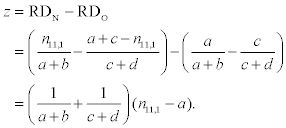Therefore, I(z) in Equation (3) can be re-expressed as I(z)=1 if n11,1 ≤ a and I(z)=0 if n11,1>a. Consequently, under the null hypothesis of n10=n01=0, the conditional exact p-value can be calculated by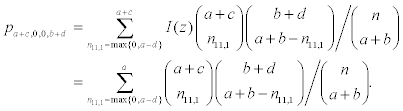This is equal to the calculation of the p-value for Fisher’s exact test. Therefore, Fisher’s exact test can be regarded as a special case of the conditional exact test given here under the null hypothesis of n10=n01=0.

Next, we show that Fisher’s exact test can be a hypothesis test for the weak causal null hypothesis when monotonicity can be assumed. The null hypothesis of n10=n01=0 implies that no subject with type (ii) or (iii) exists, and thus subjects who suffered the event are limited to those with type (i) (i.e., (Y(1), Y(0))=(1, 1)), and subjects who did not suffer the event are limited to those with type (iv) (i.e., (Y(1), Y(0))=(0, 0)). Therefore, this null hypothesis corresponds to

H0: Y(1)=Y(0) for all individuals,

which is referred to as the sharp causal null hypothesis . Clearly, whenever the sharp causal null hypothesis holds, the weak causal null hypothesis also holds. However, rejection of the sharp causal null hypothesis does not imply that the weak causal null hypothesis is rejected. This can be explained using the concept of principal stratification as discussed below, and is illustrated using hypothetical data in the following section.

As all subjects must be those with (Y(1), Y(0))=(1, 1) or (0, 0) under the sharp causal null hypothesis, rejection of this hypothesis implies that subjects with (Y(1), Y(0))=(1, 0) or (0, 1) exist. However, even when such subjects are present, if the number of subjects with (Y(1), Y(0))=(1, 0) is equal to the number with (Y(1), Y(0))=(0, 1) (> 0), the weak causal null hypothesis still cannot be rejected (i.e., we cannot deny that the true risk difference is zero), because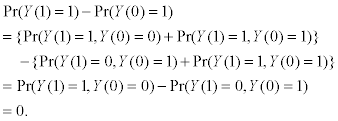Consequently, in general, Fisher’s exact test cannot be a hypothesis test for the weak causal null hypothesis.

Nevertheless, Fisher’s exact test can be a hypothesis test for the weak causal null hypothesis under the following monotonicity assumption [13,14]:

Assumption 1 (Monotonicity):

Y(0) ≤ Y(1) for all individuals.

This assumption implies that there is no subject with (Y(1), Y(0))=(0, 1). Therefore, under Assumption 1, rejection of the sharp causal null hypothesis implies that there are subjects with (Y(1), Y(0))=(1, 0). Then, the weak causal null hypothesis is also rejected, because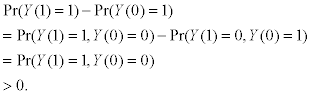This demonstrates that, under Assumption 1, whenever the sharp causal null hypothesis is rejected, the weak causal null hypothesis is also rejected. Consequently, under the monotonicity assumption, Fisher’s exact test is legitimately a hypothesis test for the weak causal null hypothesis.

Barnard’s exact test

Barnard’s exact test considers the following null hypothesis:

H0: Pr(Y=1 | X=1)=Pr(Y=1 | X=0),

where Pr(Y=1 | X=1) represents the response proportion for subjects who received the treatment, and Pr(Y=1 | X=0) represents the response proportion for subjects who received the control. Therefore, in general, the null hypothesis for Barnard’s exact test is the descriptive null hypothesis to compare two different populations , but not the causal null hypothesis to compare the different treatment statuses from one population.

Nevertheless, under randomization, two distributions generated from a single random sample may be the same as those generated by taking two independent random samples [15-17]; i.e., Pr(Y(x)=1)=Pr(Y=1 | X=x) for x=0, 1. If this is true, the following exchangeability assumption  must hold:

Assumption 2 (exchangeability):

Pr(Y(x)=1 | X=1)=Pr(Y(x)=1 | X=0) for x=0, 1.

This assumption means that for x=1, the response proportion for subjects in the treatment group is equal to that if subjects in the control group had received the treatment, and similarly for x=0, the response proportion for subjects in the control group is equal to that if subjects in the treatment group had received the control. As a hypothesis test for the causal effect, Barnard’s exact test requires the exchangeability assumption. See Greenland  for a detailed discussion.

Applying the concept of principal stratification, Assumption 2 implies that when subjects are assigned in a 1:1 ratio by randomization, the numbers of subjects with each type of (i)-(iv) in the treatment group are exactly equal to those in the control group. Although this exact equality may hold at least approximately when the sample size is very large, it may not be true when the sample size is small. For example, by chance, the numbers of subjects with type (i) and (ii) may be greater in the treatment group than in the control group, and instead the numbers of subjects with type (iii) and (iv) may be less in the treatment group than in the control group. Therefore, Assumption 2 may not strictly hold in many randomized trials, and then Barnard’s exact test, which requires this assumption, may not adequately test the causal null hypothesis to compare two different treatment statuses from one population.

However, the exact tests given here directly test the weak causal null hypothesis; they do not require that the numbers of subjects with each type of (i)-(iv) in the treatment group are equal to those in the control group when subjects are assigned in a 1:1 ratio by randomization. Therefore, the exact tests do not require Assumption 2. Rather, the exact tests yield the p-value by comparing the risk differences under the null hypothesis generated by violation of Assumption 2 with the risk difference estimated from the observed data. This violation can incidentally be caused as a result of random assignment.

In Table 4, we have summarized the assumptions and the pros and cons of the three exact tests (Fisher, Barnard, and Proposed) for the weak causal null hypothesis.

Fisher Barnard Proposed
Assumption Monotonicity Exchangeability None
Pros It is sufficient to test the sharp causal null hypothesis. It is not a problem to assume exchangeability in randomized trials. No assumption is required.
Cons Rejection of the sharp causal null hypothesis does not imply that the causal risk difference is not zero. Exchangeability may not hold in randomized trials with moderate to small samples.

Table 4: Assumptions and the pros and cons of the three exact tests (Fisher, Barnard, and Proposed) for the weak causal null hypothesis.

#### Extension to Non-Inferiority Trials and Confidence Intervals

Non-inferiority trials

The derived conditional and unconditional exact tests are extended to hypothesis testing for non-inferiority trials below. Hypothesis testing of non-inferiority focuses on the null hypothesis of Pr(Y(1)=1) - Pr(Y(0)=1)=δ rather than Pr(Y(1)=1) - Pr(Y(0)=1)=0, where δ (> 0) is a small quantity specified in advance. Again, we can express as Pr(Y(1)=1)=(n11+n10)/n and Pr(Y(0)=1)=(n11+n01)/n by using the concept of principal stratification. Therefore, the nu11 hypothesis for non-inferiority, Pr(Y(1)=1)-Pr(Y(0)=1)=δ, can be expressed as n10- n01=δn.

However, when δn is not an integer value, the null hypothesis for the exact tests cannot be prescribed. Therefore, we set the null hypothesis to a maximum integer value satisfying n10-n01 ≤ δn. Consequently, for non-inferiority trials, the conditional and unconditional exact p-values are calculated by substituting n10=n01 in the set of conditions 1 and 2 by n10-n01=m, where m is a maximum integer value satisfying m ≤ δn.

Confidence intervals

A confidence interval (CI) for a single parameter θ is defined as follows: The interval (L, U) is a 100(1-α)% CI for θ if Pr(L ≤ θ ≤ U)=1-α . The value of L can be found by seeking a minimum value of the null hypothesis that is not rejected at the significance level α/2, and similarly the value of U can be found by seeking a maximum value of the null hypothesis that is not rejected at the significance level α/2.

Since the causal risk difference can be expressed as Pr(Y(1)=1)- Pr(Y(0)=1)=(n10-n01)/n, the upper limit of 100(1 - α)% CI for the risk difference, U, linking to the unconditional exact test can be calculated as follows: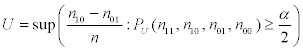where PU(n11, n10, n01, n00) is pn11 ,n10 ,n01 ,n01 in Equation (1) with set of conditions 1 excluding n10=n01, and the lower limit, L, can be calculated as follows: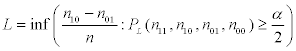where PL(n11, n10, n01, n00) is pn11 ,n10 ,n01 ,n00 in Equation (1) with set of conditions 1 excluding n10=n01, where the reverse inequality is adopted for the indicator I(z); i.e., I(z)=1 if z ≥ 0 and I(z)=0 if z<0 with z :=RDN- RDO. To derive the CI linking to the conditional exact test, Equation (2) and set of conditions 1 are replaced by Equation (3) and set of conditions 2.

It is important to note that the upper limit of this CI cannot be larger than the upper bound for the nonparametric bounds [20,21], Pr(Y = 1, X = 1) + Pr(Y = 0, X = 0), and, likewise, the lower limit cannot be smaller than the lower bound, –{Pr(Y = 1, X = 0) + Pr(Y = 0, X = 1)}, even when the sample size is very small. Therefore, the width of CI is always smaller than or equal to 1. This is because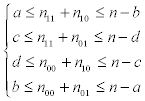which is derived from the second equation and the fourth inequality in set of conditions 1, corresponds to the nonparametric bounds:

Pr(Y=1, X=x) ≤ Pr(Y(x)=1) ≤ 1- Pr(Y=0, X=x) for x=0, 1.

#### Illustration

We illustrate the derived conditional and unconditional exact tests using the data from two clinical trials. The first is a cardiac arrest clinical trial, which is a superiority trial, and the second is an oncology clinical trial, which is a non-inferiority trial. We also show that rejection of the sharp causal null hypothesis does not imply that the weak causal null hypothesis is rejected using the data from a hypothetical clinical trial.

Application to a cardiac arrest clinical trial

Perondi et al.  reported a cardiac arrest clinical trial evaluating the next dose of epinephrine to be taken to children suffering cardiac arrest when the initial dose of epinephrine was unsuccessful. In this trial, subjects were randomly assigned in a 1:1 ratio to receive either the same (standard) dose or a higher dose. The endpoint was survival at 24 hours. The results are summarized in Table 5. The risk difference was -0.1765.

Survival at 24 hours
Group Yes No Total
Higher dose 1 33 34
Standard dose 7 27 34

Table 5: Results from a cardiac arrest clinical trial.

The unconditional exact test with r=1 yielded the two-sided p-values shown in Figure 1, with several possible combinations of (n11, n10, n01, n00). The maximum p-value was 0.0415, which was calculated under n10=n01=9. The 95% CI was -0.3382 (-23/68) to -0.0147 (-1/68). The conditional exact test yielded the two-sided p-values shown in Figure 2, with several possible combinations of (n11, n10, n01, n00). The maximum p-value was 0.0555, which was also calculated under n10=n01=9. The 95% CI was -0.3529 (-24/68) to 0 (0/68). We note that, in this example, twice the one-sided p-value was equal to the sum of the one-sided p-value and the opposite one-sided p-value.

Figure 1: Two-sided p-values for the unconditional exact test under several possible combinations of (n11, n10, n01, n00) for the data in Table 5, where the null hypothesis is n10=n01

Figure 2: Two-sided p-values for the conditional exact test under several possible combinations of (n11, n10, n01, n00) for the data in Table 5, where the null hypothesis is n10=n01.

In Figure 2, it seems that the p-value under n10=n01=0, which corresponds to Fisher’s exact test, behaves exceptionally. This is simply because Equation (3) with n10=n01=0 is more discrete than Equation (3) with n10=n01≠0. As discreteness is smaller when the sample size is larger, the extent of the exceptional behavior will be smaller with the larger sample size. Conversely, the p-value under n10=n01=0 will be largest for a small sample size, for which violation of the monotonicity assumption (i.e., that at least one subject with (Y(1), Y(0))=(0, 1) exists in the trial) will not be assured.

Application to an oncology clinical trial

Rodary et al.  reported an oncology clinical trial, a childhood nephroblastoma study, to demonstrate that pre-operative chemotherapy (new treatment) was not inferior to radiation therapy (standard treatment) in terms of tumor rupture proportions following nephrectomy. The criterion for non-inferiority required that the difference in the proportion of subjects who developed tumor rupture was 0.1 between the chemotherapy (PC) and radiation (PR) groups; i.e., the null hypothesis was PC-PR=0.1. The subjects were randomly assigned to either group in a 1:1 ratio. The results are summarized in Table 6. The risk difference was -0.0353.

Tumor rupture
Treatment Yes No Total
Chemotherapy 5 83 88
Radiation 7 69 76

Table 6: Results from an oncology clinical trial.

To apply the conditional and unconditional exact tests, we set the null hypothesis to n10-n01=16 because δn=0.1×164=16.4. The respective unconditional and conditional exact tests yielded the onesided p-values displayed in Figures 3 and 4, under several possible combinations of (n11, n10, n01, n00). The unconditional exact test yielded a maximum p-value of 0.003640 when n01=22 and 95% CI of -0.1280 (-21/164) to 0.0610 (10/164), and the conditional exact test yielded a maximum p-value of 0.003601 when n01=22 and 95% CI of -0.1280 (-21/164) to 0.0610 (10/164).

Figure 3: One-sided p-values for the unconditional exact test under several possible combinations of (n11, n10, n01, n00) for the data in Table 6, where the null hypothesis is n10-n01=16.

Figure 4: One-sided p-values for the conditional exact test under several possible combinations of (n11, n10, n01, n00) for the data in Table 6, where the null hypothesis is n10-n01=16.

A hypothetical clinical trial

To demonstrate that rejection of the sharp causal null hypothesis does not imply that the weak causal null hypothesis is rejected, we use the data from a hypothetical randomized clinical trial, shown in Table 7. The risk difference is -0.1000.

Event
Group Occurred Not occurred Total
Treatment 1 69 70
Control 8 62 70

Table 7: Results from a hypothetical clinical trial.

The conditional exact test for the null hypothesis of n10=n01 yielded the one-sided p-values shown in Figure 5, with several possible combinations of (n11, n10, n01, n00). The maximum p-value was 0.0371 under n10=n01=26, which corresponds to the p-value for the weak causal null hypothesis. Under n10=n01=0, which corresponds to the p-value for the sharp causal null hypothesis, the p-value was 0.0166. At the significance level of 0.025 (one-sided), the sharp null hypothesis is rejected but the weak causal null hypothesis is not rejected.

Figure 5: One-sided p-values for the conditional exact test under several possible combinations of (n11, n10, n01, n00) for the data in Table 7, where the null hypothesis is n10=n01.

As noted in the above cardiac arrest clinical trial, the extent of the exceptional behavior of the p-value under n10=n01=0 will decrease with a larger sample size. This is demonstrated by comparison of Figures 2 and 5. For the larger sample size, there will be more cases in which the sharp null hypothesis is rejected, but the weak causal null hypothesis is not rejected.

#### Discussion and Conclusion

In this article, we have derived conditional and unconditional exact tests for the weak causal null hypothesis on a binary outcome in randomized trials, using the concept of principal stratification. The derived exact tests have the advantage that they can be extended to non-inferiority trials and to construct CIs in a straightforward manner as a unified approach.

The unconditional exact test will be applied to randomized trials with complete (or equally simple) randomization, and the conditional exact test will be applied to randomized trials with any restriction. However, restricted randomization does not randomly select all a+b subjects of n subjects, and some of them are assigned with dependence on already assigned subjects. Therefore, the conditional exact test may strictly be invalid under restricted randomization. This problem was also pointed out in the context of Fisher’s exact test .

It might be thought that the exact tests given here should be compared with the existing exact tests for numerical aspects. However, such comparisons would be meaningless, because our new exact tests are hypothesis tests for the weak causal null hypothesis, whereas existing exact tests are not. It is important to consider which null hypothesis should be tested. In many randomized trials, this will be the weak causal null hypothesis H0: Pr(Y(1)=1)=Pr(Y(0)=1). As an example, let us consider the cardiac arrest clinical trial illustrated in this article. In this trial, researchers set the sample size under the assumption that the 24-hour survival proportion would increase from 20% in the standard dose group to 50% in the higher dose group. This assumption implies that there would certainly be subjects with type 10 (n10 ≠ 0). However, one cannot deny that the 24-hour survival proportion would be higher in the standard dose group than in the higher dose group (i.e., that there would be subjects with type 01 (n01 ≠ 0)). Therefore, at the time of study planning, we should determine to test the weak causal null hypothesis rather than the sharp causal null hypothesis, which corresponds to the null hypothesis of n10=n01=0.

The derived exact tests require a numerical search to yield the p-value for the hypothesis testing. The computational effort increases dramatically with the sample size. Therefore, further work is needed to create an efficient algorithm with which the derived exact tests will be feasible. Recently, Rigdon and Hudgens  reported a method to construct the CIs applying a similar, but different, approach. Further work will be to compare their CI method with ours.

#### Acknowledgement

The author thanks the reviewers for helpful comments. This work was supported partially by Grant-in-Aid for Scientific Research (No. 15K00057) from Japan Society for the Promotion of Science.

#### References

Select your language of interest to view the total content in your interested language

### Article Usage

• Total views: 12609
• [From(publication date):
August-2015 - Aug 19, 2019]
• Breakdown by view type
• HTML page views : 8786
• PDF downloads : 3823

## Post your commentCan't read the image? click here to refresh
###### Peer Reviewed Journals

Make the best use of Scientific Research and information from our 700 + peer reviewed, Open Access Journals

International Conferences 2019-20

Meet Inspiring Speakers and Experts at our 3000+ Global Annual Meetings

Top